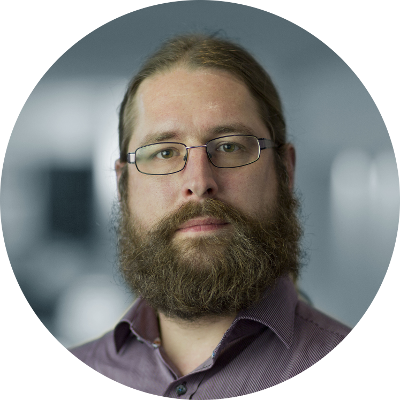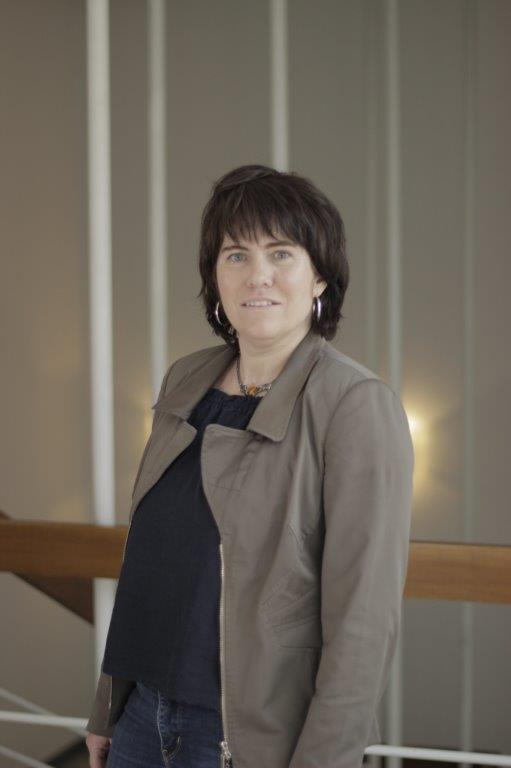# Our Institute

Institute of Applied Analysis and Numerical Simulation

Structure of the Institute.

The Institute of Applied Analysis and Numerical Simulation comprises five Research Groups.

Many processes in natural and engineering sciences are mathematically described by systems of nonlinear partial differential equations. Any approach towards a deeper understanding and simulation of these processes requires a close interplay between mathematical modelling, analysis and numerics. The group aims at major contributions to these topics in an interdisciplinary context. A particular focus is on problems in mathematical fluid mechanics that lead to systems of conservation laws. Applications include multiscale and multiphysics problems in compressible fluid flow, multiphase flow in free and porous media domains, radiation- and magnetohydrodynamics as well as particle flow.

The group bridges the gap between Numerical Mathematics and Computational Science: "Hardware-oriented numerics" aims at including hardware aspects directly into the design of new methods. One central focus is on iterative linear and nonlinear finite element multigrid and domain decomposition methods, for massively parallel HPC systems and heterogeneous machines including accelerator hardware. Besides classical runtime and scalability, this mandates methodological progress in fault tolerance, communication avoidance , asynchrony and adaptive floating point schemes. In various interdisciplinary collaborations, the methods are applied to seismic wave propagation and seismic imaging, to multiscale forward and inverse biomechanical problems, and to signal propagation in multimode fibres.

Efficient discretizations and solvers for partial differential equations (PDE's) and eigenvalue problems

Key aspects of our research are efficient numerical methods for the approximation of (non-)linear partial differential equations and eigenvalue problems, and their efficient implementation with applications in computational chemistry and physics.

The group investigates and develops methods for efficient and certified numerical simulation by surrogate modelling. In particular the focus is put on model reduction (reduced basis methods for parametric problems) and kernel methods (data-based modelling for high-dimensional function approximation, data-analysis, machine learning). The resulting models are used in optimization, control, inverse problems or uncertainty quantification. Software-development is an accompanying effort. Application fields comprise flow and transport-problems, porous media, heterogeneous domain decomposition problems, biomechanics, elastic multibody systems, soft-tissue-robotics or pervasive computing.

Mathematical models to describe natural phenomena are often based on partial differential equations. The inputs to the equation, such as coefficients, boundary or initial conditions, the data or the domain itself may not be known a-priori. Insufficient or imprecise measurements have to be accounted for when the model is supposed to be realistic. The equation itself could, further, be chaotic. Stochastic partial differential equations account for all sorts of uncertainties and chaotic behaviour. The aim of the group is to investigate solution concepts for stochastic partial differential equations, analyse solutions and invent numerical methods to approximate solutions, hence, bridge the gap between stochastic analysis and numerical simulations. The methods developed have applications in various natural and engineering sciences.### Dominik Göddeke

Prof. Dr. rer. nat.